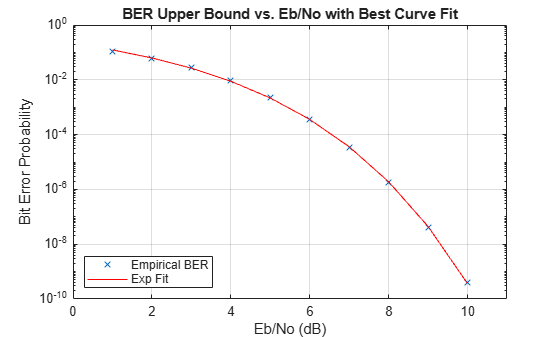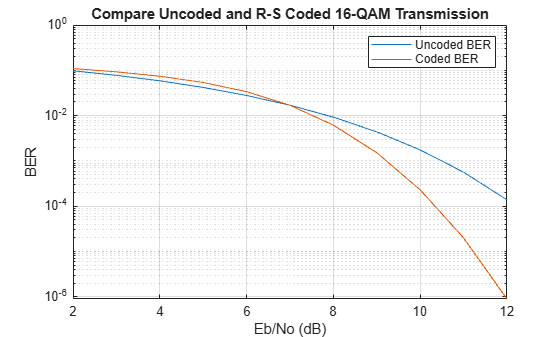# bercoding

Bit error rate (BER) for coded AWGN channels

## Syntax

```berub = bercoding(EbNo,'conv',decision,coderate,dspec) berub = bercoding(EbNo,'block','hard',n,k,dmin) berub = bercoding(EbNo,'block','soft',n,k,dmin) berapprox = bercoding(EbNo,'Hamming','hard',n) berub = bercoding(EbNo,'Golay','hard',24) berapprox = bercoding(EbNo,'RS','hard',n,k) berapprox = bercoding(...,modulation) ```

## Description

`berub = bercoding(EbNo,'conv',decision,coderate,dspec) ` returns an upper bound or approximation on the BER of a binary convolutional code with coherent phase shift keying (PSK) modulation over an additive white Gaussian noise (AWGN) channel. `EbNo` is the ratio of bit energy to noise power spectral density, in dB. If `EbNo` is a vector, `berub` is a vector of the same size, whose elements correspond to the different Eb/N0 levels. To specify hard-decision decoding, set `decision` to `'hard'`; to specify soft-decision decoding, set `decision` to `'soft'`. The convolutional code has code rate equal to `coderate`. The `dspec` input is a structure that contains information about the code's distance spectrum:

• `dspec.dfree` is the minimum free distance of the code.

• `dspec.weight` is the weight spectrum of the code.

To find distance spectra for some sample codes, use the `distspec` function or see  and .

### Note

The results for binary PSK and quadrature PSK modulation are the same. This function does not support M-ary PSK when M is other than 2 or 4.

`berub = bercoding(EbNo,'block','hard',n,k,dmin) ` returns an upper bound on the BER of an [`n`,`k`] binary block code with hard-decision decoding and coherent BPSK or QPSK modulation. `dmin` is the minimum distance of the code.

`berub = bercoding(EbNo,'block','soft',n,k,dmin) ` returns an upper bound on the BER of an [`n`,`k`] binary block code with soft-decision decoding and coherent BPSK or QPSK modulation. `dmin` is the minimum distance of the code.

`berapprox = bercoding(EbNo,'Hamming','hard',n) ` returns an approximation of the BER of a Hamming code using hard-decision decoding and coherent BPSK modulation. (For a Hamming code, if n is known, then k can be computed directly from n.)

`berub = bercoding(EbNo,'Golay','hard',24) ` returns an upper bound of the BER of a Golay code using hard-decision decoding and coherent BPSK modulation. Support for Golay currently is only for n=24. In accordance with , the Golay coding upper bound assumes only the correction of 3-error patterns. Even though it is theoretically possible to correct approximately 19% of 4-error patterns, most decoders in practice do not have this capability.

`berapprox = bercoding(EbNo,'RS','hard',n,k) ` returns an approximation of the BER of (n,k) Reed-Solomon code using hard-decision decoding and coherent BPSK modulation.

`berapprox = bercoding(...,modulation)` returns an approximation of the BER for coded AWGN channels when you specify a `modulation` type. See the `berawgn` function for a listing of the supported modulation types.

## Examples

collapse all

Find an upper bound on the theoretical BER of a (23,12) block code.

Set the example parameters.

```n = 23; % Codeword length k = 12; % Message length dmin = 7; % Minimum distance EbNo = 1:10; % Eb/No range (dB)```

Estimate the BER.

`berBlk = bercoding(EbNo,'block','hard',n,k,dmin);`

Plot the estimated BER.

```berfit(EbNo,berBlk) ylabel('Bit Error Probability') title('BER Upper Bound vs. Eb/No, with Best Curve Fit')```Estimate the performance of a 16-QAM channel in AWGN when encoded with a (15,11) Reed-Solomon code using hard-decision decoding.

Set the input Eb/No range and determine the uncoded BER for 16-QAM.

```ebno = (2:12)'; uncodedBER = berawgn(ebno,'qam',16);```

Estimate the coded BER for 16-QAM channel with a (15,11) Reed-Solomon code using hard decision decoding.

`codedBER = bercoding(ebno,'RS','hard',15,11,'qam',16);`

Plot the estimated BER curves.

```semilogy(ebno,[uncodedBER codedBER]) grid legend('Uncoded BER','Coded BER') xlabel('Eb/No (dB)') ylabel('BER')```## Limitations

The numerical accuracy of this function's output is limited by

• Approximations in the analysis leading to the closed-form expressions that the function uses

• Approximations related to the numerical implementation of the expressions

You can generally rely on the first couple of significant digits of the function's output.

## Alternatives

As an alternative to the `bercoding` function, invoke the BERTool GUI (`bertool`) and use the Theoretical tab.

## References

 Proakis, J. G., Digital Communications, 4th ed., New York, McGraw-Hill, 2001.

 Frenger, P., P. Orten, and T. Ottosson, “Convolutional Codes with Optimum Distance Spectrum,” IEEE Communications Letters, Vol. 3, No. 11, Nov. 1999, pp. 317–319.

 Odenwalder, J. P., Error Control Coding Handbook, Final Report, LINKABIT Corporation, San Diego, CA, 1976.

 Sklar, B., Digital Communications, 2nd ed., Prentice Hall, 2001.

 Ziemer, R. E., and R. L., Peterson, Introduction to Digital Communication, 2nd ed., Prentice Hall, 2001.